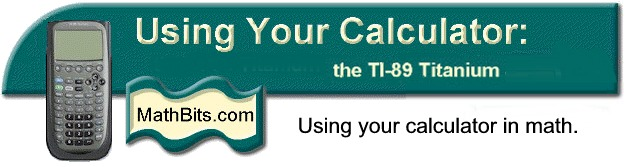Working with Derivatives
on the TI-89 Titanium
 Differentiate

The differentiate command,
d ( differentiate),
computes derivatives.  It is located in the F3 Calc menu.  It is also available directly from the face of the keyboard above the 8 key (2nd 8).
Be careful!!! The letter D on the keyboard is not the same.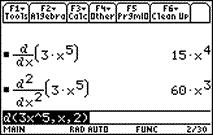To get the second derivative, enter
d (3x^5,x,2).  You can also take the derivative of a previous answer with
d(ans(1),x) where you recall the answer.
To get an antiderivative (without constant) enter
d
(3x^5,x,-1).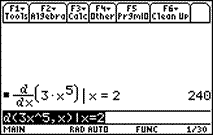To evaluate a derivative at a specific value, use with.  The with bar ( | ) is located:
TI-89:  its own key on the left
• Remember:  You must ALWAYS specify the variable parameter.  The calculator must know with respect to which variable you are differentiating.  This is true even if there is only one variable in the problem.

 nDeriv

The nDeriv command computes numerical derivatives.
It is located in the F3 Calc menu as #A nDerive.

You will not need to type the capital D if you type in this command.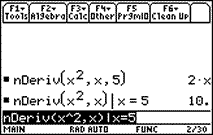To evaluate the numerical derivative at a number, use the with bar.

Not as useful as the exact derivative.

The formula used by the calculator to generate nDeriv(f(x),x) is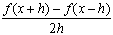where h is 0.001 unless otherwise specified.Return to Open Page for TI-89 Titanium